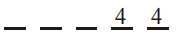# In a random experiment,Question:

In a random experiment, a fair die is rolled until two fours are obtained in succession. The probability that the experiment will end in the fifth throw of the die is equal to:

1. $\frac{150}{6^{5}}$

2. $\frac{175}{6^{5}}$

3. $\frac{200}{6^{5}}$

4. $\frac{225}{6^{5}}$

Correct Option: , 2

Solution:$\frac{1}{6^{2}}\left(\frac{5^{3}}{6^{3}}+\frac{2 C_{1} \cdot 5^{2}}{6^{3}}\right)=\frac{175}{6^{5}}$